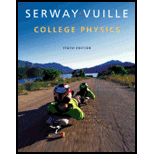# An AC source operating at 60. Hz with a maximum voltage of 170 V is connected in series with a resistor ( R = 1.2 kΩ) and an inductor ( L = 2.8 H). (a) What is the maximum value of the current in the circuit? (b) What are the maximum values of the potential difference across the resistor and the inductor? (c) When the current is at a maximum, what are the magnitudes of the potential differences across the resistor, the inductor, and the AC source? (d) When the current is zero, what are the magnitudes of the potential difference across the resistor, the inductor, and the AC source?### College Physics

10th Edition
Raymond A. Serway + 1 other
Publisher: Cengage Learning
ISBN: 9781285737027

#### Solutions

Chapter
Section### College Physics

10th Edition
Raymond A. Serway + 1 other
Publisher: Cengage Learning
ISBN: 9781285737027
Chapter 21, Problem 24P
Textbook Problem
1 views

## An AC source operating at 60. Hz with a maximum voltage of 170 V is connected in series with a resistor (R = 1.2 kΩ) and an inductor (L = 2.8 H). (a) What is the maximum value of the current in the circuit? (b) What are the maximum values of the potential difference across the resistor and the inductor? (c) When the current is at a maximum, what are the magnitudes of the potential differences across the resistor, the inductor, and the AC source? (d) When the current is zero, what are the magnitudes of the potential difference across the resistor, the inductor, and the AC source?

(a)

To determine
The maximum current in the circuit.

### Explanation of Solution

Given Info: The resistance of the resistor is 1.2 . The inductance of the inductor is 2.8H . The frequency of the ac circuit is 60Hz . Maximum voltage of the source is 170V .

The inductive reactance of the circuit is,

XL=2πfL

• f is the frequency of the ac source
• L is the inductance of the inductor

Substitute 60Hz for f , 2.8H for L to determine the inductive reactance,

XL=2π(60Hz)(2.8H)=1.1×103Ω

The formula to calculate the impedance of the circuit where the components are in series is given by,

Z=R2+(XLXC)2

• R is the resistance of the resistor
• XL is the inductive reactance
• XC is the capacitive reactance

Substitute 1.2 for R , 1.1×103Ω for XL , 0 for XC to determine the impedance of the circuit,

Z=(1

b)

To determine
The maximum voltage across the resistor and inductor.

c)

To determine
The potential difference across the resistor, inductor, and the ac source when the current is at its maximum.

d)

To determine
The potential difference across the resistor, inductor, and the ac source when the current is at it is zero.

### Still sussing out bartleby?

Check out a sample textbook solution.

See a sample solution

#### The Solution to Your Study Problems

Bartleby provides explanations to thousands of textbook problems written by our experts, many with advanced degrees!

Get Started

Find more solutions based on key concepts
Platinum has a density of 21.4 g/cm3. What is the mass of 5.9 cm3 of this metal?

General Chemistry - Standalone book (MindTap Course List)

Photosynthesis runs on the energy of ___________. a. light b. hydrogen ions c. O2 d. CO2

Biology: The Unity and Diversity of Life (MindTap Course List)

Fried banana or vegetable snack chips make a healthy everyday snack choice for vegetarians. T F

Nutrition: Concepts and Controversies - Standalone book (MindTap Course List)

Why does nuclear fusion in the sun occur only near the center?

Horizons: Exploring the Universe (MindTap Course List)

How can errors in the cell cycle lead to cancer in humans?

Human Heredity: Principles and Issues (MindTap Course List)

Write the correct IUPAC name for each of the following: a. b. c. d. e.

Chemistry for Today: General, Organic, and Biochemistry

An uncharged capacitor and a resistor are connected in series to a source of emf. If = 9.00 V, C = 20.0 F, and...

Physics for Scientists and Engineers, Technology Update (No access codes included)

How does the oceans density stratification limit the vertical movement of seawater?

Oceanography: An Invitation To Marine Science, Loose-leaf Versin